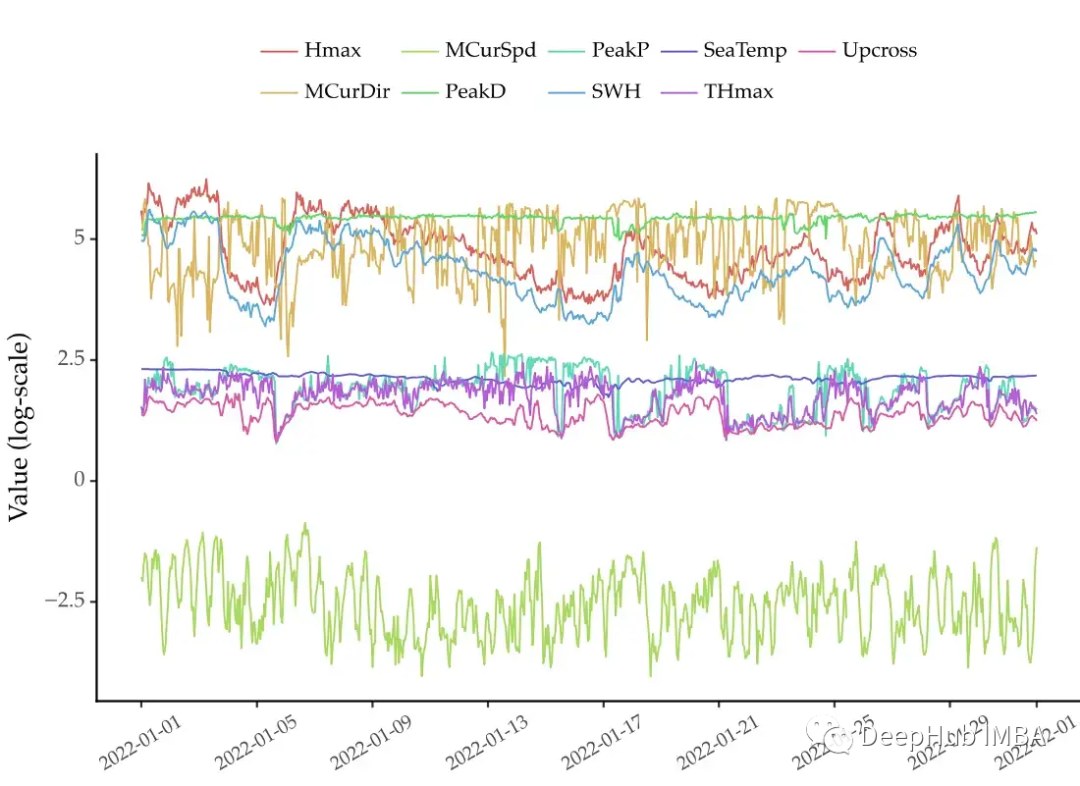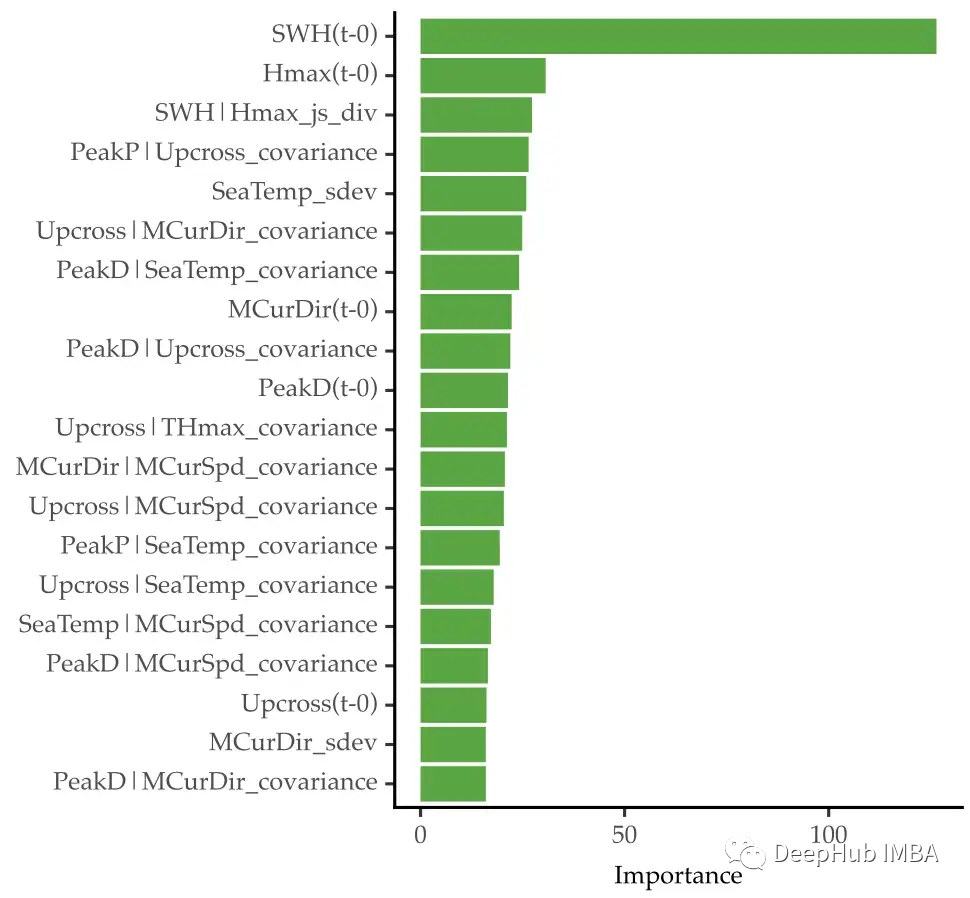0

# 多元时间序列特征工程的指南

## 简介## 基线模型

`````` import pandas as pd

# skipping second row, setting time column as a datetime column
# dataset available here: https://github.com/vcerqueira/blog/tree/main/data
skiprows=,
parse_dates=['time'])

# setting time as index
buoy.set_index('time', inplace=True)
# resampling to hourly data
buoy = buoy.resample('H').mean()
# simplifying column names
buoy.columns = [
'PeakP', 'PeakD', 'Upcross',
'SWH', 'SeaTemp', 'Hmax', 'THmax',
'MCurDir', 'MCurSpd'
]
``````

`````` import pandas as pd

from sklearn.model_selection import train_test_split
from sklearn.metrics import mean_absolute_percentage_error as mape
from sklearn.multioutput import MultiOutputRegressor
from lightgbm import LGBMRegressor

# https://github.com/vcerqueira/blog/blob/main/src/tde.py
from src.tde import time_delay_embedding

target_var = 'SWH'

colnames = buoy.columns.tolist()

# create data set with lagged features using time delay embedding
buoy_ds = []
for col in buoy:
col_df = time_delay_embedding(buoy[col], n_lags=24, horizon=12)
buoy_ds.append(col_df)

# concatenating all variables
buoy_df = pd.concat(buoy_ds, axis=1).dropna()

# defining target (Y) and explanatory variables (X)
predictor_variables = buoy_df.columns.str.contains('\(t\-')
target_variables = buoy_df.columns.str.contains(f'{target_var}\(t\+')
X = buoy_df.iloc[:, predictor_variables]
Y = buoy_df.iloc[:, target_variables]

# train/test split
X_tr, X_ts, Y_tr, Y_ts = train_test_split(X, Y, test_size=0.3, shuffle=False)

# fitting a lgbm model without feature engineering
model_wo_fe = MultiOutputRegressor(LGBMRegressor())
model_wo_fe.fit(X_tr, Y_tr)

# getting forecasts for the test set
preds_wo_fe = model_wo_fe.predict(X_ts)

# computing the MAPE error
mape(Y_ts, preds_wo_fe)
# 0.238
``````

## 多元时间序列的特征工程

• 单变量特征提取。计算各变量的滚动统计。例如，滚动平均可以用来消除虚假的观测;
• 二元特征提取。计算变量对的滚动统计，以总结它们的相互作用。例如，两个变量之间的滚动协方差。

`````` import numpy as np

SUMMARY_STATS = {
'mean': np.mean,
'sdev': np.std,
}

univariate_features = {}
# for each column in the data
for col in colnames:
# get lags for that column
X_col = X.iloc[:, X.columns.str.startswith(col)]

# for each summary stat
for feat, func in SUMMARY_STATS.items():
# compute that stat along the rows
univariate_features[f'{col}_{feat}'] = X_col.apply(func, axis=1)

# concatenate features into a pd.DF
univariate_features_df = pd.concat(univariate_features, axis=1)
``````

• 滚动二元统计。计算以变量对作为输入的统计信息。例如，滚动协方差或滚动相关性滚动二元统计的例子包括协方差、相关性或相对熵。
• 滚动二元变换，然后单变量统计。这将一对变量转换为一个变量，并对该变量进行统计。例如，计算元素相互关系，然后取其平均值。有许多二元转换的方法。例如，百分比差异、相互关联或成对变量之间的线性卷积。通过第一步操作后，用平均值或标准偏差等统计数据对这些转换进行汇总。

`````` import itertools

import pandas as pd

from scipy.spatial.distance import jensenshannon
from scipy import signal
from scipy.special import rel_entr

from src.feature_extraction import covariance, co_integration

BIVARIATE_STATS = {
'covariance': covariance,
'co_integration': co_integration,
'js_div': jensenshannon,
}

BIVARIATE_TRANSFORMATIONS = {
'corr': signal.correlate,
'conv': signal.convolve,
'rel_entr': rel_entr,
}

# get all pairs of variables
col_combs = list(itertools.combinations(colnames, 2))

bivariate_features = []
# for each row
for i, _ in X.iterrows():
# feature set in the i-th time-step
feature_set_i = {}
for col1, col2 in col_combs:
# features for pair of columns col1, col2

# getting the i-th instance for each column
x1 = X.loc[i, X.columns.str.startswith(col1)]
x2 = X.loc[i, X.columns.str.startswith(col2)]

# compute each summary stat
for feat, func in BIVARIATE_SUMMARY_STATS.items():
feature_set_i[f'{col1}|{col2}_{feat}'] = func(x1, x2)

# for each transformation
for trans_f, t_func in BIVARIATE_TRANSFORMATIONS.items():

# apply transformation
xt = t_func(x1, x2)

# compute summary stat
for feat, s_func in SUMMARY_STATS.items():
feature_set_i[f'{col1}|{col2}_{trans_f}_{feat}'] = s_func(xt)

bivariate_features.append(feature_set_i)

bivariate_features_df = pd.DataFrame(bivariate_features, index=X.index)
``````

`````` # concatenating all features with lags
X_with_features = pd.concat([X, univariate_features_df, bivariate_features_df], axis=1)

# train/test split
X_tr, X_ts, Y_tr, Y_ts = train_test_split(X_with_features, Y, test_size=0.3, shuffle=False)

# fitting a lgbm model with feature engineering
model_w_fe = MultiOutputRegressor(LGBMRegressor())
model_w_fe.fit(X_tr, Y_tr)

# getting forecasts for the test set
preds_w_fe = model_w_fe.predict(X_ts)

# computing MAPE error
print(mape(Y_ts, preds_w_fe))
# 0.227
``````

## 特征选择

`````` # getting the importance of each feature in each horizon
avg_imp = pd.DataFrame([x.feature_importances_
for x in model_w_fe.estimators_]).mean()

# getting the top 100 features
n_top_features = 100

importance_scores = pd.Series(dict(zip(X_tr.columns, avg_imp)))
top_features = importance_scores.sort_values(ascending=False)[:n_top_features]
top_features_nm = top_features.index

# subsetting training and testing sets by those features
X_tr_top = X_tr[top_features_nm]
X_ts_top = X_ts[top_features_nm]

# re-fitting the lgbm model
model_top_features = MultiOutputRegressor(LGBMRegressor())
model_top_features.fit(X_tr_top, Y_tr)

# getting forecasts for the test set
preds_top_feats = model_top_features.predict(X_ts_top)

# computing MAE error
mape(Y_ts, preds_top_feats)
# 0.229
``````## 总结

• 多变量时间序列预测通常是一个自回归过程
• 特征工程是数据科学项目中的一个关键步骤。
• 可以用特征工程改进多元时间序列数据。这包括计算单变量和双变量转换和汇总统计信息。
• 提取过多的特征会导致高维问题。可以使用特征选择方法来删除不需要的特征。

### “多元时间序列特征工程的指南”的评论:

##### 关于作者##### Deephub